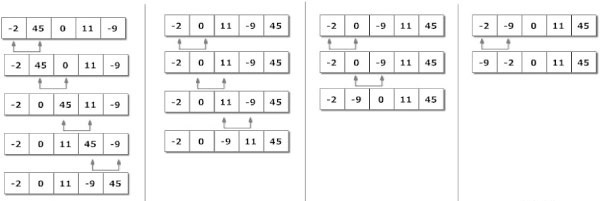Data Structure

# Introduction

Bubble Sort is a simple sorting algorithm that works by repeatedly stepping through the list to be sorted, comparing each pair of adjacent items and swapping them if they are in the wrong order.

### Algorithm Of Bubble Sort

```1. Each two adjacent elements are compared
2. Swap with  lighter elements
3. Move forward and swap with each lighter item
4. If there is a heavier element, then this item begins to bubble to the surface
5. Finally the Heaviest Element is on its place
```### Implementation Of Bubble Sort

```#include <stdio.h>
#include <conio.h>
void main( )
{
int arr ;
int i, j, n,temp ;
clrscr();
printf("Enter the size of array:");
scanf("%d",&n);
printf("\nEnter the item in array:\n");
for(i = 0 ; i < n ; i++)
{
scanf("%d",&arr[i]);
}
for ( i = 0 ; i < n ; i++ )
{
for ( j = 0 ; j <= n - i ; j++ )
if ( arr[j] > arr[j + 1] )
temp = arr[j] ;
arr[j] = arr[j + 1] ;
arr[j + 1] = temp ;
}
printf ( "\nArray after sorting:") ;
for ( i = 0 ; i < n ; i++ )
printf ( "%d\t", arr[i] ) ;
getch();
}
```